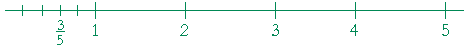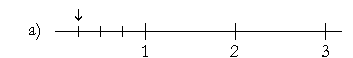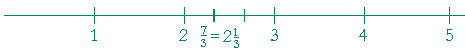PROPER FRACTIONS, MIXED NUMBERS, IMPROPER FRACTIONS

PROBLEMS

Do the problem yourself first!

 11.  In this fraction 34 what does the denominator signify and what does

11. the numerator?

The denominator signifies that number 1 has been divided into 4 equal parts. The numerator signifies that we are counting 3 of them.

 11.  Place 34 on this number line. (How many time must you cut the line?)11.  To divide a line into four equal parts -- that is, into fourths -- cut it three times.

Why is that number called "three-fourths"?

Because 3 is three fourths of 4.

 12.  a)  Why is this number 13 called "one-third"?

Because 1 is one third of 3.

 12.  b) 13 is which part of 1?  One third.
 12.  c)  Place 13 on this number line.13.  What ratio has each number to 1?

 13.  a) 12 is one half of 1. b) 14 is one quarter of 1. 12.  c) 34 is three quarters of 1. d) 13 is one third of 1. 13.  e) 23 is two thirds of 1. f) 58 is five eighths of 1.

 14.  a) 7 12 Seven twelfths. b) 11 2 Eleven halves. 14.  c) 83 Eight thirds. d) 3759 37 over 59. 14.  e) 9 100 9 hundredths. f) 7   1000 7 thousandths.
 14.  g) 246     1,000,000 246 millionths.
 14.  h)  6 38 Six and three eighths. i)  7 516 Seven and five sixteenths.

15.  Write the meaning of each mixed number.

 14.     a)  1 12 = 1 + 12 b)  2 34 = 2 + 34 c)  3 58 = 3 + 58

16.  From this list, pick out the proper fractions, and pick out the
16.  improper fractions.

 25 52 79 97 77 78 85

Proper fractions:

 25 79 78

Improper fractions:

 52 97 77 85

17.  What kind of fractions are less than 1?  Proper fraction.

 1 8.  a)   What number is three fifths of 1? 35

18.  b)  Place that number on the number line.19.  What number is at the arrow?14452 23

10.  Cut the number line and place each number on it:

 15 25 35 45 1 25 2 15 4 3511.  Cut the number line and place each number on it.

 a) 23b) 34c) 58d)  2 34e)  3 3812.  Answer with a mixed number or with a whole number plus a
12.  remainder
, whichever makes sense.

12.  a)  It takes three yards of material to make a skirt. How many skirts
12.  a)  can you make from 25 yards?

8 skirts. 1 yard will remain.

11.  b)  You are going on a journey of 25 miles, and you have gone a
11.  b)  third of the distance. How far have you gone?

 8 13 miles.

13.  Change each improper fraction to a mixed number or a whole
13.  number.  Do not write the division box.  Divide mentally.

 12.   a) 75 = 1 25 b) 24 3 = 8 c) 23 9 = 2 59 d) 31 8 = 3 78 12.   e) 1010 = 1 f) 1212 = 1 g) 71 9 = 7 89 h) 53 6 = 8 56 12.   i) 63 7 = 9 j) 43 6 = 7 16 k) 75 8 = 9 38 l) 74 9 = 8 29

14.  Change each mixed number to an improper fraction.

 11.     a)  1 23 = 53 b)  3 25 = 17 5 c)  7 58 = 61 8 d)  9 67 = 69 7 14.     e)  6 59 = 59 9 f)  8 47 = 60 7 g)  7 16 = 43 6 h)  9 78 = 79 8

15.  Place on the number line.

 15.   a) 7315.   b) 15 414.   c) 23 516.  How much is a third of 7?

 73 = 2 13

To take a third of a number, divide by 3.

Continue on to Section 2:  Comparing fractions.

or